close

## Thank you for your enquiry!

One of our training experts will be in touch shortly to go over your training requirements.close

Press esc to closeBack to course information

## Thank you for your enquiry!

One of our training experts will be in touch shortly to go overy your training requirements.## Thank you for your enquiry!

One of our training experts will be in touch shortly to go over your training requirements.

## Course Information

### Probability and Statistics for Data Science​ Training Course Outline

The following modules will be covered during this Probability and Statistics for Data Science Course:

Module 1: Basic Probability Theory

• Probability Spaces
• Conditional Probability
• Independence

Module 2: Random Variables

• What are Random Variables?
• Discrete Random Variables
• Continuous Random Variables
• Conditioning on an Event
• Functions of Random Variables
• Generating Random Variables

Module 3: Multivariate Random Variables

• Introduction to Multivariate Random Variables
• Discrete Random Variables
• Continuous Random Variables
• Joint Distributions of Discrete and Continuous Variables
• Independence
• Generating Multivariate Random Variables
• Rejection Sampling

Module 4: Expectation

• Expectation Operator
• Mean and Variance
• Covariance
• Conditional Expectation

Module 5: Random Processes

• Introduction to Random Process
• Mean and Autocovariance Functions
• Independent Identically-Distributed Sequences Gaussian Process
• Poisson Process
• Random Walk

Module 6: Convergence of Random Processes

• Types of Convergence
• Law of Large Numbers
• Central Limit Theorem
• Monte Carlo Simulation

Module 7: Markov Chains

• Markov Property
• Time-Homogeneous Discrete-Time Markov Chains
• Recurrence
• Periodicity
• Convergence
• Markov-Chain Monte Carlo

Module 8: Descriptive Statistics

• Introduction to Descriptive Statistics
• Types of Descriptive Statistics

Module 9: Frequentist Statistics

• Mean Square Error
• Consistency
• Conﬁdence Intervals
• Nonparametric Model Estimation
• Parametric Model Estimation
• Maximum Likelihood

Module 10: Bayesian Statistics

• Bayesian Parametric Models
• Conjugate Prior
• Bayesian Estimators

Module 11: Hypothesis Testing

• Hypothesis-Testing Framework
• Parametric Testing
• Nonparametric Testing: The Permutation Test
• Multiple Testing

Module 12: Linear Regression

• Introduction to Linear Regression
• Linear Models
• Least-Squares Estimation

Show morePrerequisites

There are no formal prerequisites for attending this Probability and Statistics for Data Science​ Training course.

Audience

This training is suitable for anyone who wants to learn how to apply probability and statistics in Data Science.

.

### Probability and Statistics for Data Science​ Training Course Overview

Probability and Statistics is the mathematical field that includes the gathering, examination, interpretation, and presentation of numerical data, which are concerned with the rules regulating random events. Statistical methods primarily depend on probability theory and make estimates for further analysis. This training assists organisations in applying ideas of randomness, prediction, expected value, and estimation with a more logical and mathematical approach. This training aims to teach probability and statistical best practices for analysing data and deriving meaningful insights from raw and unstructured data. Pursuing this Probability and Statistics for Data Science Training course will allow individuals to handle an investigation of data and advance their careers in Data Science.

In this 2-day Probability and Statistics for Data Science​ Training course, delegates will gain a thorough understanding of applying probability theory and statistics in Data Science. During this training, they will learn about conditional probability to measure in the probability space, which contains the intersection of the sets. They will also learn about the joint distributions of discrete and continuous random variables that define the probability of two events. Our highly skilled tutor with years of teaching experience will conduct this course and help delegates comprehend probability theory.

Course Objectives

• To represent and analyse uncertain phenomena using a framework
• To quantify the outcome of the experiment as belonging to a specific event
• To assign probabilities to each occurrence of interest and an experiment
• To become accustomed to Markov chains and different statistical types
• To generate samples from the appropriate conditional distribution
• To evaluate the occurrence of a particular event that influences another event

After completing this course, delegates will be able to apply probability theory in data science using random variables. They will also be able to define a valid probability measure on the power set of R and integrate density to obtain the probability of the random variables.

Show moreWhy choose us

## Ways to take this course

Our easy to use Virtual platform allows you to sit the course from home with a live instructor. You will follow the same schedule as the classroom course, and will be able to interact with the trainer and other delegates.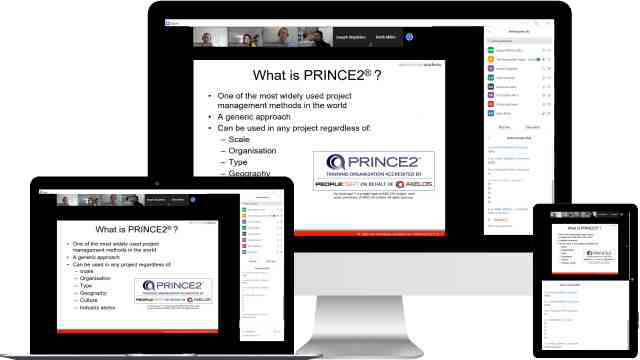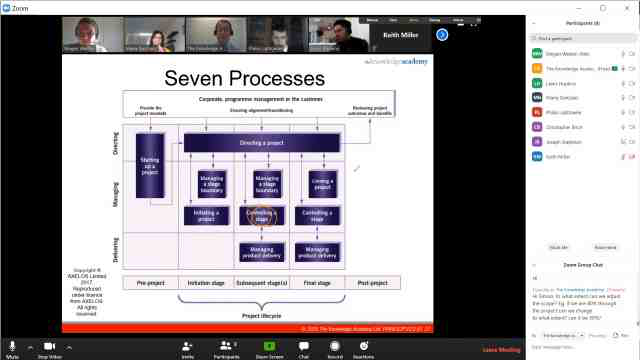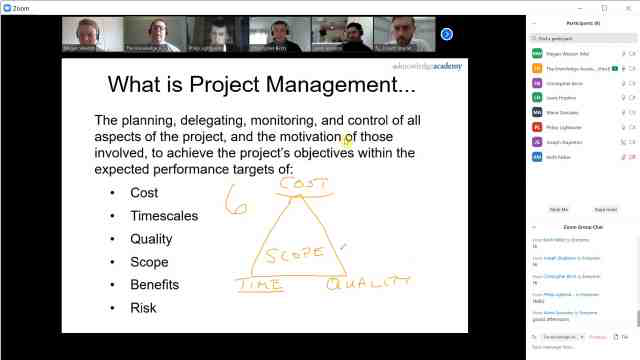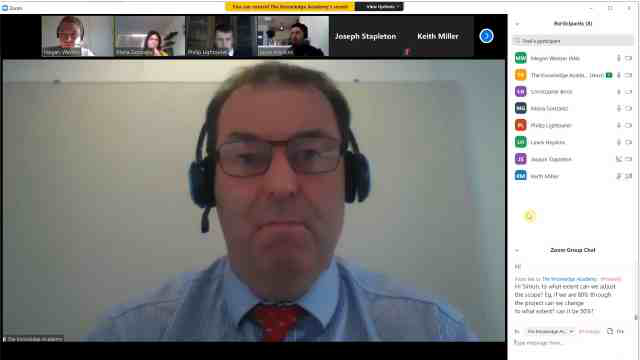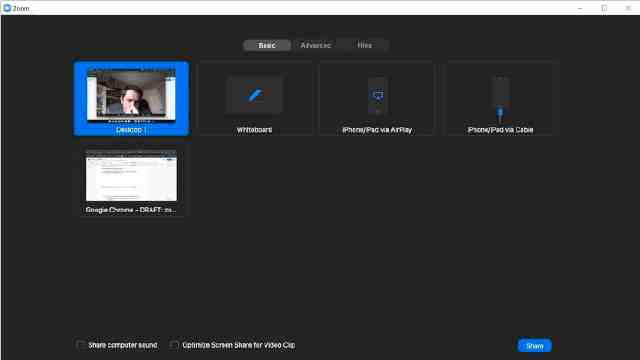Our fully interactive online training platform is compatible across all devices and can be accessed from anywhere, at any time. All our online courses come with a standard 90 days access that can be extended upon request. Our expert trainers are constantly on hand to help you with any questions which may arise.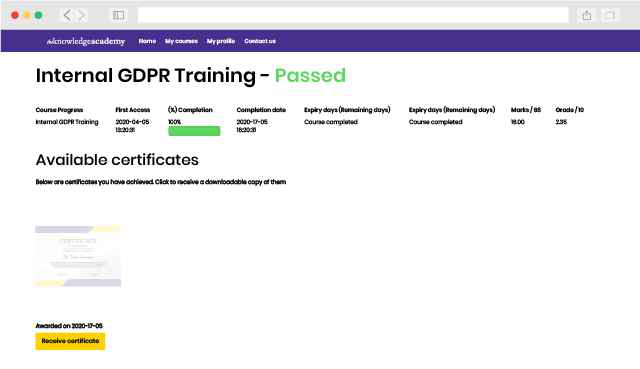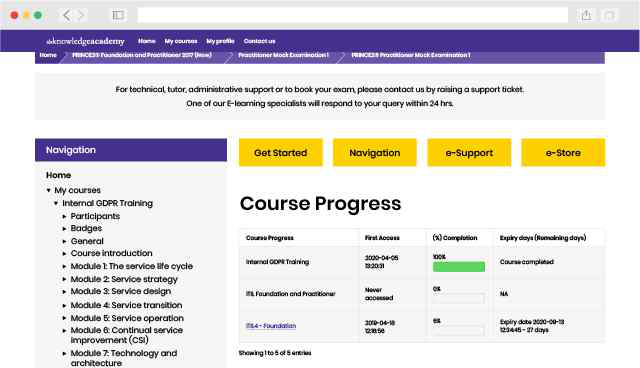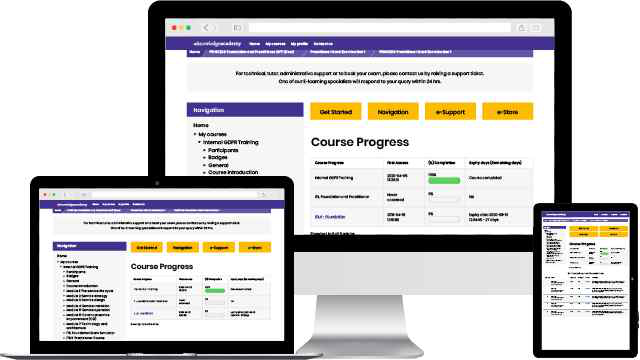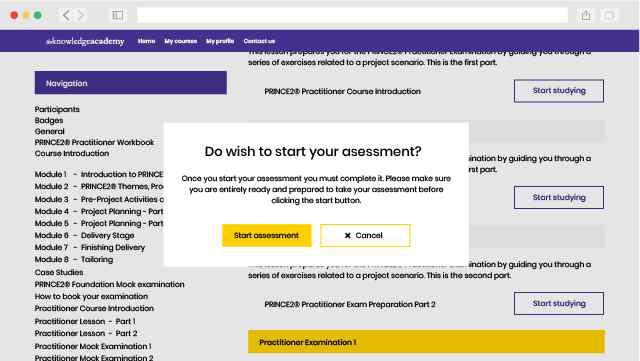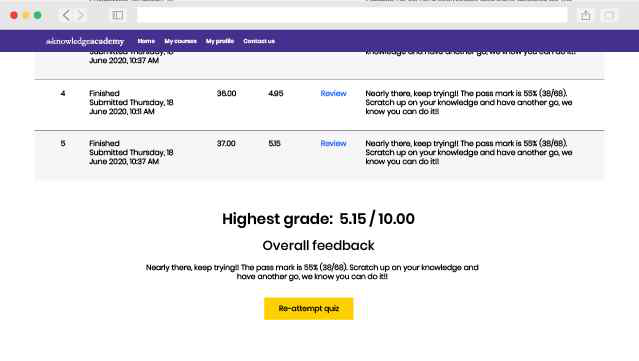## Probability and Statistics for Data Science Training FAQs

### FAQ's

#### What is the use of probability and statistics in Data Science?

Probability is used to predict the outcome of an event about to occur, and statistics is helpful to estimate the values for further analysis, which depends on probability theory. Both probability and statistics rely on the data.

#### What are the pre-requisites?

There are no formal prerequisites for attending this Probability and Statistics for Data Science Training course.

#### Who should attend this course?

This training is suitable for anyone who wants to learn how to apply probability and statistics in Data Science.

#### What is the Markov Chain?

Markov Chain is the mathematical function used to model random processes in discrete spaces that satisfy the Markov property. Markov property is satisfied when a current state can predict a future state.

#### What is a random variable?

A random variable is a numeric value associated with a probability that depends on the random process. It can be discrete or continuous.

#### What is the duration of this course?

This course is 2 days.

#### What is hypothesis testing?

Hypothesis testing is the statistical testing of the predictions made by Researchers and Data Scientists regarding the natural world, whether these are true or not.

#### What will I learn in this Probability and Statistics for Data Science Training course?

In this Probability and Statistics for Data Science Training course, you will learn how to apply probability theory and statistics in data science for prediction and estimations, hypothesis testing, Markov Chain, linear regression, multivariate random variables, expectations, random processes, descriptive statistics, and other related concepts.

#### What is the cost/training fees for Probability and Statistics for Data Science Training certification in Canada?

The price for Probability and Statistics for Data Science Training certification in Canada starts from CAD3095

#### Which is the best training institute/provider of Probability and Statistics for Data Science Training in Canada?

The Knowledge Academy is the Leading global training provider for Probability and Statistics for Data Science Training.

## Why choose us### Best price in the industry

You won't find better value in the marketplace. If you do find a lower price, we will beat it.### Many delivery methods

Flexible delivery methods are available depending on your learning style.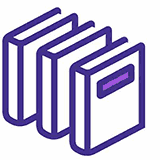### High quality resources

Resources are included for a comprehensive learning experience.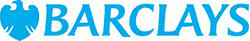"Really good course and well organised. Trainer was great with a sense of humour - his experience allowed a free flowing course, structured to help you gain as much information & relevant experience whilst helping prepare you for the exam"

Joshua Davies, Thames WaterBack to course information